Courses

# Surveying 3 MCQ

## 20 Questions MCQ Test Mock test series of SSC JE Civil Engineering | Surveying 3 MCQ

Description
This mock test of Surveying 3 MCQ for Civil Engineering (CE) helps you for every Civil Engineering (CE) entrance exam. This contains 20 Multiple Choice Questions for Civil Engineering (CE) Surveying 3 MCQ (mcq) to study with solutions a complete question bank. The solved questions answers in this Surveying 3 MCQ quiz give you a good mix of easy questions and tough questions. Civil Engineering (CE) students definitely take this Surveying 3 MCQ exercise for a better result in the exam. You can find other Surveying 3 MCQ extra questions, long questions & short questions for Civil Engineering (CE) on EduRev as well by searching above.
QUESTION: 1

### A scale of 1 inch = 50 ft. is mentioned on an old map. What is the corresponding equivalent scale?

Solution:

1 feet = 12 inches

1 inch = 2.54 cm

50 feet = 12 x 50 x 2.54 cm = 1524 cm = 15.24 m

So, 2.54 cm = 15.24 m

Hence 1 cm = 6 m

QUESTION: 2

Solution:
QUESTION: 3

### Identify the incorrect statement:

Solution:

Bench mark is a permanent reference point whose R.L is always known to us.

It is our first step to adjust the level for its temporary adjustment (centering, levelling) before start to take reading from a level.

Change point is a point which denotes shifting of level either due to inability to take reading or due to some obstruction.

QUESTION: 4

An object on the top of a hill 100 m high is just visible above the horizon from a station at sea level. The distance between the station and the object is:

Solution:

Distance between the station and the object is given by: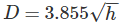Where,

h = height of visible horizon (meters)

D = Distance of visible horizon (km)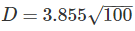D = 38.53 km.

QUESTION: 5

If every one of the three angles of a triangle have a probable error of ±1′′, then what will be the probable error in the sum of the measured internal angles of the triangle?

Solution:

Let probable error in angle “A” is = σA = ±1′′

probable error in angle “B” is = σB = ±1′′

Probable error in angle “C” is = σC = ±1′′

Probable error in the sum of the measured internal angles of the triangle is: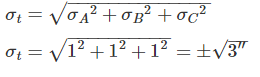QUESTION: 6

Direct ranging is possible only when the end stations are:

Solution:

The process of establishing or developing intermediate points between two terminal points or end points on a straight line is known as ranging.

Direct Ranging: The ranging in which intermediate ranging rods are placed in a straight line by direct observation from either end.

Direct ranging is possible only when the end stations are intervisible.

QUESTION: 7

Which type of errors introduces due to clogging of chain rings with mud?

Solution:

Due to clogging of chain rings with mud, actual length of the chain will become less than nominal length of chain. Therefore, measured value is greater than true value.

Error in measurement = Measured value – True value

Error = +ve

QUESTION: 8

If whole circle bearing of any line is W1, that of the preceding line is W2 and “d” is the deflection angles to the right, then choose the correct expression:

Solution:
QUESTION: 9

The back sight reading on bench mark of R.L 500.00 m is 2.685 m. If foresight reading on the point is 1.345 m, the reduced level of the point is?

Solution:
QUESTION: 10

The following consecutive readings were taken with a dumpy level and a 3 m staff on a continuous sloping ground:

0.425, 1.035, 1.950, 2.360, 2.950, 0.750, 1.565, 2.455.

Which of the following readings are back sights?

Solution:
QUESTION: 11

A correction for error due to refraction is:

Solution:
QUESTION: 12

If cross sectional area of an embankment at 30 m intervals are 20, 40, 60, 50 and 30 m2 respectively, then volume of the embankment on the basis of prismoidal rule is:

Solution:

Volume of embankment is given by: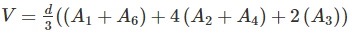d = 30 m

A1 = 20 m2

A2 = 40 m2

A3 = 60 m2

A= 50 m2

A= 30 m2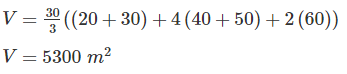QUESTION: 13

To orient a plane table at a point with two inaccessible points, the method generally adopted is:

Solution:

Two Point Problem: It is basically a resection method. In the two-point problem, two points are sighted from other point corresponding to the points given in plane table sheet.

In the method of intersection, the plotted position of stations is known, and the plotted position of objects are obtained by intersection.

In the method of resection, the plotted position of objects is known, and the plotted position of station is obtained.

QUESTION: 14

The indirect method of contouring has all the following advantages over direct method except:

Solution:

Direct Method v/s Indirect Method of contouring: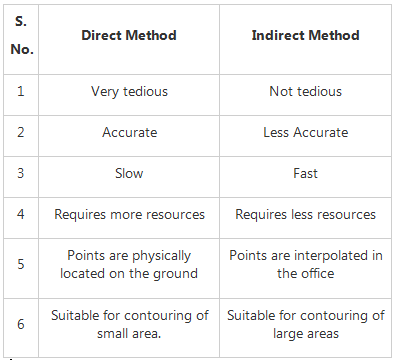QUESTION: 15

The values of whole circle bearing vary from ________.

Solution:

The values of the whole circle Bearing varies from 0° to 360° and is always taken with respect to North.

QUESTION: 16

If the degree of curve is 1, then radius of the curve for 30 m chain is

Solution:
QUESTION: 17

A dumpy level is set up with its eyepiece vertically over a peg A. The height from the top of peg A to the centre of the eyepiece is 1.540 m and the reading on peg B is 0.705 m. The level is then set up over B. The height of the eyepiece above peg B is 1.490 m and a reading on A is 2.195 m. The difference in level between A and B is ________.

Solution:
QUESTION: 18

A level when set up 25 m from peg A and 50 m from peg B reads 2.847 m on staff held on A and 3.462 m on staff held on B, keeping bubble at its centre while reading. If the reduced levels of A and B are 283.665 m and 284.295 m respectively, what is the collimation error per 100.0 m?

Solution: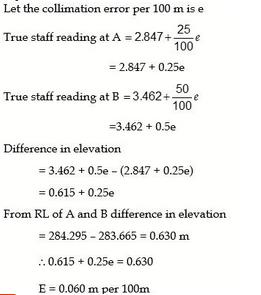QUESTION: 19

A 30 m metric chain is found to be 20 cm too long throughout a measurement. If the distance measured is recorded as 300 m, what is the actual distance?

Solution:

True length of chain × true measurement = incorrect length of chain × incorrect measurement

Hence, True measurement =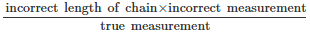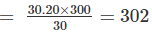QUESTION: 20

The difference between face left and face right observation of a theodolite is 3’. The error is:

Solution:

Error is half the difference between the face left and face right observation.

Error = 3'/2 = 1'30"